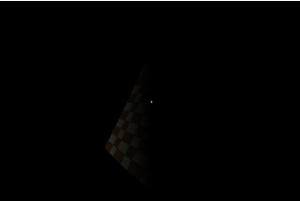modern opengl tutorial

end

# 第十八课 漫反射光

## 背景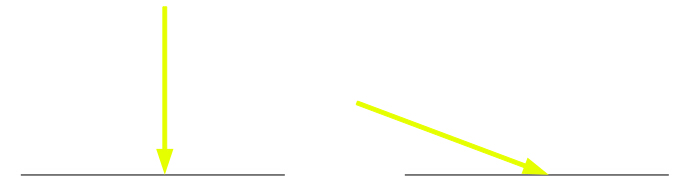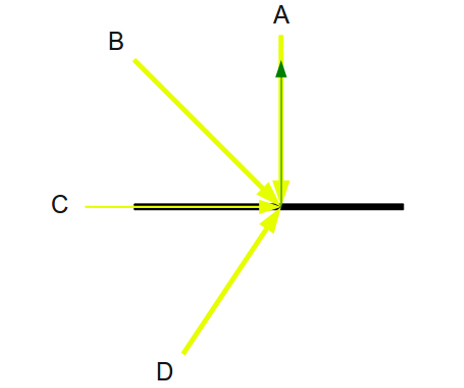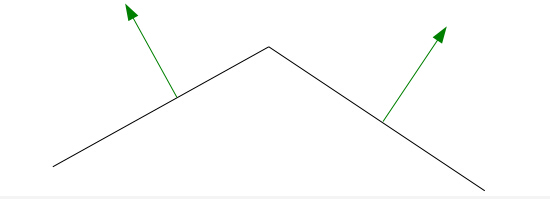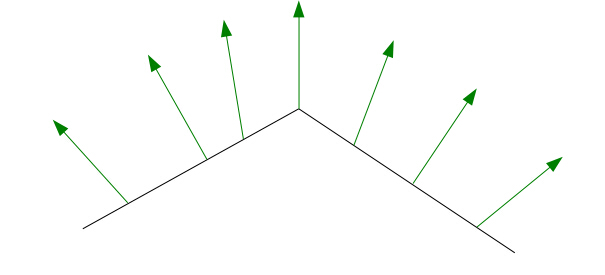## 代码

``````[cpp] view plaincopy
(lighting_technique.h:25)
struct DirectionalLight
{
Vector3f Color;
float AmbientIntensity;
Vector3f Direction;
float DiffuseIntensity;
};  ``````

``````[cpp] view plaincopy
(lighting.vs)
/#version 330
layout (location = 0) in vec3 Position;
layout (location = 1) in vec2 TexCoord;
layout (location = 2) in vec3 Normal;
uniform mat4 gWVP;
uniform mat4 gWorld;
out vec2 TexCoord0;
out vec3 Normal0;
void main()
{
gl_Position = gWVP * vec4(Position, 1.0);
TexCoord0 = TexCoord;
Normal0 = (gWorld * vec4(Normal, 0.0)).xyz;
}  ``````

``````[cpp] view plaincopy
/#version 330
in vec2 TexCoord0;
in vec3 Normal0;
out vec4 FragColor;
struct DirectionalLight
{
vec3 Color;
float AmbientIntensity;
float DiffuseIntensity;
vec3 Direction;
};  ``````

``````(lighting.fs:19)
void main()
{
vec4 AmbientColor =vec4(gDirectionalLight.Color, 1.0f) *  gDirectionalLight.AmbientIntensity;``````

``    Float DiffuseFactor = dot(normalize(Normal0),-gDirectionalLight.Direction);``

1. 顶点着色器传来的法线数据在使用前需要标准化。这是因为向量经过插值后其模可能会被改变而不再是一个单位向量。
2. 光的方向被反转。
3. 光向量没有被标准化。为所有的像素重复标准化相同向量将是对 GPU 资源的浪费。相反，我们只用确保程序传来向量在绘制命令调用之前已被标准化。
``````vec4 DiffuseColor;
if (DiffuseFactor > 0) {
DiffuseColor = vec4(gDirectionalLight.Color, 1.0f) * gDirectionalLight.DiffuseIntensity * DiffuseFactor;
}
else {
DiffuseColor = vec4(0, 0, 0, 0);
}``````

``````    FragColor = texture2D(gSampler, TexCoord0.xy) * (AmbientColor + DiffuseColor);
}``````

``````[cpp] view plaincopy
(lighting_technique.cpp:144)
void LightingTechnique::SetDirectionalLight(const DirectionalLight& Light)
{
glUniform3f(m_dirLightLocation.Color, Light.Color.x, Light.Color.y, Light.Color.z);
glUniform1f(m_dirLightLocation.AmbientIntensity, Light.AmbientIntensity);
Vector3f Direction = Light.Direction;
Direction.Normalize();
glUniform3f(m_dirLightLocation.Direction, Direction.x, Direction.y, Direction.z);
glUniform1f(m_dirLightLocation.DiffuseIntensity, Light.DiffuseIntensity);
}  ``````

``````[cpp] view plaincopy
(tutorial18.cpp:35)
struct Vertex
{
Vector3f m_pos;
Vector2f m_tex;
Vector3f m_normal;
Vertex() {}
Vertex(Vector3f pos, Vector2f tex)
{
m_pos = pos;
m_tex = tex;
m_normal = Vector3f(0.0f, 0.0f, 0.0f);
}
}; ``````

``````[cpp] view plaincopy
(tutorial18.cpp:197)
void CalcNormals(const unsigned int* pIndices, unsigned int IndexCount, Vertex* pVertices, unsigned int VertexCount)
{
for (unsigned int i = 0 ; i < IndexCount ; i += 3) {
unsigned int Index0 = pIndices[i];
unsigned int Index1 = pIndices[i + 1];
unsigned int Index2 = pIndices[i + 2];
Vector3f v1 = pVertices[Index1].m_pos - pVertices[Index0].m_pos;
Vector3f v2 = pVertices[Index2].m_pos - pVertices[Index0].m_pos;
Vector3f Normal = v1.Cross(v2);
Normal.Normalize();
pVertices[Index0].m_normal += Normal;
pVertices[Index1].m_normal += Normal;
pVertices[Index2].m_normal += Normal;
}
for (unsigned int i = 0 ; i < VertexCount ; i++) {
pVertices[i].m_normal.Normalize();
}
}  ``````

``````[cpp] view plaincopy
</pre><pre name="code" class="cpp">(tutorial18.cpp:131)
const Matrix4f& WorldTransformation = p.GetWorldTrans();
m_pEffect->SetWorldMatrix(WorldTransformation);
...
glEnableVertexAttribArray(2);
...
glVertexAttribPointer(2, 3, GL_FLOAT, GL_FALSE, sizeof(Vertex), (const GLvoid*)20);
...
glDisableVertexAttribArray(2);  ``````

## 操作结果